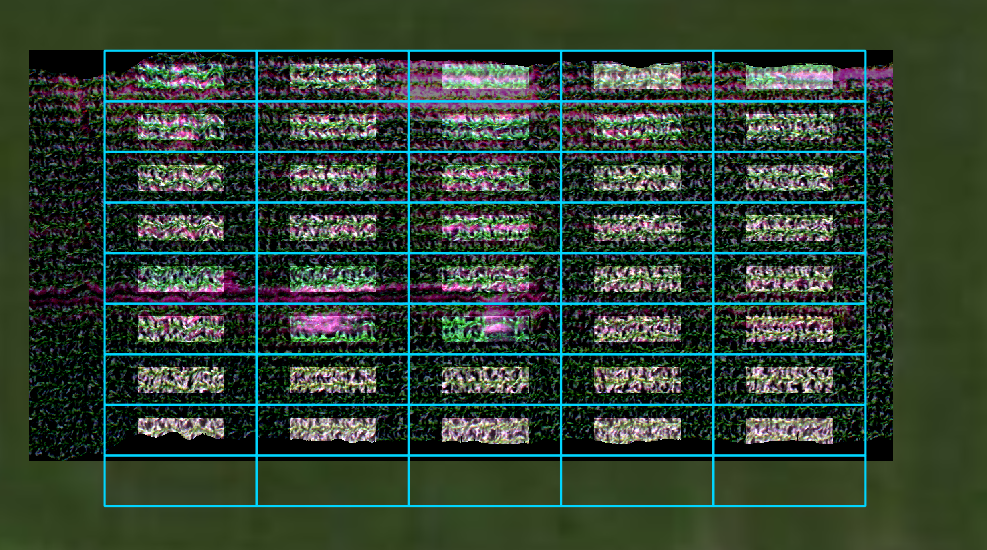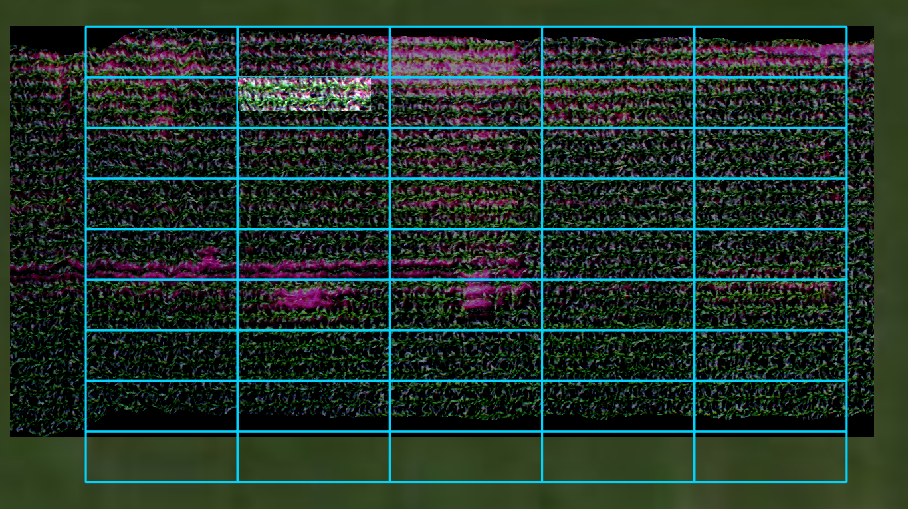# 8.1.6. spatial_mod¶

class `hs_process.``spatial_mod`(spyfile, gdf=None)[source]

Bases: `object`

Class for manipulating data within the spatial domain (e.g., cropping a datacube by a geographical boundary).

Methods Summary

 `crop_many_gdf`([spyfile, gdf, plot_id_ref, …]) Crops many plots from a single image by comparing the image to a `crop_single`([pix_e_ul, pix_n_ul, …]) Crops a single plot from an image. `load_spyfile`(spyfile) Loads a `SpyFile` (Spectral Python object) for data access and/or manipulation by the `hstools` class.

Methods Documentation

`crop_many_gdf`(spyfile=None, gdf=None, plot_id_ref=None, pix_e_ul=None, pix_n_ul=None, n_plots=None)[source]

Crops many plots from a single image by comparing the image to a polygon file (`geopandas.GoeDataFrame`) that contains plot information and geometry of plot boundaries.

Parameters:
spyfile (`SpyFile` object, optional): The datacube to crop; if

`None`, loads datacube and band information from `spatial_mod.spyfile` (default: `None`).

gdf (`geopandas.GeoDataFrame`, optional): the plot IDs and

polygon geometery of each of the plots; ‘plot’ must be used as a column name to identify each of the plots, and should be an integer; if `None`, loads geodataframe from `spatial_mod.gdf` (default: `None`).

plot_id_ref (`int`, optional): the plot ID of the reference plot.

`plot_id_ref` is required if passing `pix_e_ul`, `pix_n_ul`, or `n_plots` because it is used as the reference point for any of the adjustments/modifications dictated by said parameters. `plot_id_ref` must be present in the `gdf`, and the extent of `plot_id_ref` must intersect the extent of the datacube (default: `None`).

pix_e_ul (`int`, optional): upper left pixel column (easting) of

`plot_id_ref`; this is used to calculate the offset between the GeoDataFrame geometry and the approximate image georeference error (default: `None`).

pix_n_ul (`int`, optional): upper left pixel row (northing) of

`plot_id_ref`; this is used to calculate the offset between the GeoDataFrame geometry and the approximate image georeference error (default: `None`).

n_plots (`int`, optional): number of plots to crop, starting with

`plot_id_ref` and moving from West to East and North to South. This can be used to limit the number of cropped plots (default; `None`).

Returns:
`pandas.DataFrame`:
• df_plots (`pandas.DataFrame`) – data for which to crop each plot; includes ‘plot_id’, ‘pix_e_ul’, and ‘pix_n_ul’ columns. This data can be passed to `spatial_mod.crop_single` to perform the actual cropping.

Note:

If `pix_e_ul` or `pix_n_ul` are passed, the pixel offset from the northwest corner of `plot_id_ref` will be calculated. This offset is then applied to all plots within the extent of the image to systematically shift the actual upper left pixel locations for each plot, effectively shifting the easting and/or northing of the upper left pixel of the hyperspectral datacube to match that of the `gdf`. If the shift should only apply to a select number of plots, `n_plots` can be passed to restrict the number of plots that are processed.

Note:

Either the pixel coordinate or the map unit coordinate should be passed for `crop_X_Y` and `buf_X_Y` in each direction (i.e., easting and northing). Do not pass both.

Example:

Load the `hsio` and `spatial_mod` modules

```>>> import geopandas as gpd
>>> import os
>>> from hs_process import hsio
>>> from hs_process import spatial_mod
```

Read datacube and spatial plot boundaries

```>>> fname_in = r'F:\nigo0024\Documents\hs_process_demo\Wells_rep2_20180628_16h56m_pika_gige_7-Convert Radiance Cube to Reflectance from Measured Reference Spectrum.bip.hdr'
>>> fname_gdf = r'F:\nigo0024\Documents\hs_process_demo\plot_bounds_small\plot_bounds.shp'
>>> io = hsio(fname_in)
>>> my_spatial_mod = spatial_mod(io.spyfile)
>>> dir_out = os.path.join(io.base_dir, 'spatial_mod', 'crop_many_gdf')
>>> name_append = '-crop-many-gdf'
```

Get instructions on how plots should be cropped via `spatial_mod.crop_many_gdf()`; note that a `pandas.DataFrame` is returned with information describing how each plot should be cropped.

```>>> df_plots = my_spatial_mod.crop_many_gdf(spyfile=io.spyfile, gdf=gdf)
>>> df_plots
plot_id  pix_e_ul  pix_n_ul  crop_e_pix  crop_n_pix
0      1018       478         0         229          76
1       918       707         0         229          76
2       818       936         0         229          76
3       718      1165         0         229          76
4       618      1394         0         229          76
5      1017       478        76         229          76
6       917       707        76         229          76
7       817       936        76         229          76
8       717      1165        76         229          76
9       617      1394        76         229          76
...
```

Use the data from the first frow of df_plots to crop a single plot from the original image (uses spatial_mod.crop_single)

```>>> pix_e_ul=113
>>> pix_n_ul=0
>>> crop_e_pix=229
>>> crop_n_pix=75
>>> plot_id=1018
pix_e_ul=pix_e_ul, pix_n_ul=pix_n_ul, crop_e_pix=crop_e_pix, crop_n_pix=crop_n_pix,
spyfile=io.spyfile, plot_id=plot_id)
```

Save the cropped datacube and geotiff to a new directory

```>>> fname_out = os.path.join(dir_out, io.name_short + '_plot_' + str(1018) + name_append + '.' + io.defaults.envi_write.interleave)
>>> fname_out_tif = os.path.join(dir_out, io.name_short + '_plot_' + str(1018) + '.tif')
Saving F:
```
igo0024Documentshs_process_demospatial_modcrop_many_gdfWells_rep2_20180628_16h56m_pika_gige_7_plot_1018-crop-many-gdf.bip

Either projection_out is None or geotransform_out is None (or both are). Retrieving projection and geotransform information by loading hsio.fname_in via GDAL. Be sure this is appropriate for the data you are trying to write.

Using a for loop, use `spatial_mod.crop_single` and `hsio.write_cube` to crop by plot and save cropped datacubes to file

```>>> for idx, row in df_plots.iterrows():
name_plot=row['plot_id'],
name_short=io.name_short)
pix_e_ul=row['pix_e_ul'], pix_n_ul=row['pix_n_ul'],
crop_e_pix=row['crop_e_pix'], crop_n_pix=row['crop_n_pix'],
buf_e_m=2.0, buf_n_m=0.75,
plot_id=row['plot_id'])
>>>     fname_out = os.path.join(dir_out, io.name_short + '_plot_' + str(row['plot_id']) + name_append + '.bip.hdr')
>>>     fname_out_tif = os.path.join(dir_out, io.name_short + '_plot_' + str(row['plot_id']) + '.tif')
>>>     io.write_cube(fname_out, array_crop, metadata=metadata, force=True)  # force=True to overwrite the plot_1018 image
Saving F:\nigo0024\Documents\hs_process_demo\crop_many_gdf\Wells_rep2_20180628_16h56m_pika_gige_7_plot_1018.bip
Saving F:\nigo0024\Documents\hs_process_demo\crop_many_gdf\Wells_rep2_20180628_16h56m_pika_gige_7_plot_918.bip
Saving F:\nigo0024\Documents\hs_process_demo\crop_many_gdf\Wells_rep2_20180628_16h56m_pika_gige_7_plot_818.bip
Saving F:\nigo0024\Documents\hs_process_demo\crop_many_gdf\Wells_rep2_20180628_16h56m_pika_gige_7_plot_718.bip
Saving F:\nigo0024\Documents\hs_process_demo\crop_many_gdf\Wells_rep2_20180628_16h56m_pika_gige_7_plot_618.bip
Saving F:\nigo0024\Documents\hs_process_demo\crop_many_gdf\Wells_rep2_20180628_16h56m_pika_gige_7_plot_1017.bip
Saving F:\nigo0024\Documents\hs_process_demo\crop_many_gdf\Wells_rep2_20180628_16h56m_pika_gige_7_plot_917.bip
Saving F:\nigo0024\Documents\hs_process_demo\crop_many_gdf\Wells_rep2_20180628_16h56m_pika_gige_7_plot_817.bip
Saving F:\nigo0024\Documents\hs_process_demo\crop_many_gdf\Wells_rep2_20180628_16h56m_pika_gige_7_plot_717.bip
Saving F:\nigo0024\Documents\hs_process_demo\crop_many_gdf\Wells_rep2_20180628_16h56m_pika_gige_7_plot_617.bip
...
```

Open cropped geotiff images in QGIS to visualize the extent of the cropped images compared to the original datacube and the plot boundaries (the full extent image is darkened and displayed in the background:`crop_single`(pix_e_ul=0, pix_n_ul=0, crop_e_pix=None, crop_n_pix=None, crop_e_m=None, crop_n_m=None, buf_e_pix=None, buf_n_pix=None, buf_e_m=None, buf_n_m=None, spyfile=None, plot_id=None, gdf=None, name_append='spatial-crop-single')[source]

Crops a single plot from an image. If `plot_id` and `gdf` are explicitly passed (i.e., they will not be loaded from `spatial_mod` class), the “map info” tag in the metadata will be adjusted to center the cropped area within the appropriate plot geometry.

Parameters
• pix_e_ul (`int`, optional) – upper left pixel column (easting) to begin cropping (default: 0).

• pix_n_ul (`int`, optional) – upper left pixel row (northing) to begin cropping (default: 0).

• crop_e_m (`float`, optional) – length of each row (easting direction) of the cropped image in map units (e.g., meters; default: `None`).

• crop_n_m (`float`, optional) – length of each column (northing direction) of the cropped image in map units (e.g., meters; default: `None`)

• crop_e_pix (`int`, optional) – number of pixels in each row in the cropped image (default: `None`).

• crop_n_pix (`int`, optional) – number of pixels in each column in the cropped image (default: `None`).

• buf_e_m (`float`, optional) – The buffer distance in the easting direction (in map units; e.g., meters) to be applied after calculating the original crop area; the buffer is considered after `crop_X_m` / `crop_X_pix`. A positive value will reduce the size of `crop_X_m` / `crop_X_pix`, and a negative value will increase it (default: `None`).

• buf_n_m (`float`, optional) – The buffer distance in the northing direction (in map units; e.g., meters) to be applied after calculating the original crop area; the buffer is considered after `crop_X_m` / `crop_X_pix`. A positive value will reduce the size of `crop_X_m` / `crop_X_pix`, and a negative value will increase it (default: `None`).

• buf_e_pix (`int`, optional) – The buffer distance in the easting direction (in pixel units) to be applied after calculating the original crop area (default: `None`).

• buf_n_pix (`int`, optional) – The buffer distance in the northing direction (in pixel units) to be applied after calculating the original crop area (default: `None`).

• spyfile (`SpyFile` object or `numpy.ndarray`) – The datacube to crop; if `numpy.ndarray` or `None`, loads band information from `self.spyfile` (default: `None`).

• plot_id (`int`) – the plot ID of the area to be cropped (default: `None`).

• gdf (`geopandas.GeoDataFrame`) – the plot names and polygon geometery of each of the plots; ‘plot’ must be used as a column name to identify each of the plots, and should be an integer. `gdf` must be explicitly passed to

• name_append (`str`) – NOT YET SUPPORTED; name to append to the filename (default: ‘spatial-crop-single’).

Returns

2-element `tuple` containing

• array_crop (`numpy.ndarray`): Cropped datacube.

• metadata (`dict`): Modified metadata describing the cropped hyperspectral datacube (`array_crop`).

Example

Load and initialize the `hsio` and `spatial_mod` modules

```>>> from hs_process import hsio
>>> from hs_process import spatial_mod
>>> fname_in = r'F:\nigo0024\Documents\hs_process_demo\Wells_rep2_20180628_16h56m_pika_gige_7-Convert Radiance Cube to Reflectance from Measured Reference Spectrum.bip.hdr'
>>> io = hsio(fname_in)
>>> my_spatial_mod = spatial_mod(io.spyfile)
```

Crop an area with a width (easting) 200 pixels and a height (northing) of 50 pixels, with a northwest/upper left origin at the 342nd column (easting) and 75th row (northing).

```>>> pix_e_ul = 342
>>> pix_n_ul = 75
>>> array_crop, metadata = my_spatial_mod.crop_single(pix_e_ul, pix_n_ul, crop_e_pix=200, crop_n_pix=50)
```

Save as a geotiff using `io.write_tif`, then load into QGIS to visualize.

```>>> fname_tif = r'F:\nigo0024\Documents\hs_process_demo\spatial_mod\crop_single\crop_single.tif'
Either `projection_out` is `None` or `geotransform_out` is `None` (or both are). Retrieving projection and geotransform information by loading `hsio.fname_in` via GDAL. Be sure this is appropriate for the data you are trying to write.
```

Open cropped geotiff image in QGIS to visualize the extent of the cropped image compared to the original datacube and the plot boundaries (the full extent image is darkened and displayed in the background):`load_spyfile`(spyfile)[source]

Loads a `SpyFile` (Spectral Python object) for data access and/or manipulation by the `hstools` class.

Parameters

spyfile (`SpyFile` object) – The datacube being accessed and/or manipulated.

Example

Load and initialize the `hsio` and `spatial_mod` modules

```>>> from hs_process import hsio
>>> from hs_process import spatial_mod
>>> fname_in = r'F:\nigo0024\Documents\hs_process_demo\Wells_rep2_20180628_16h56m_pika_gige_7-Convert Radiance Cube to Reflectance from Measured Reference Spectrum.bip.hdr'
>>> io = hsio(fname_in)
>>> my_spatial_mod = spatial_mod(io.spyfile)
```

Load datacube using `spatial_mod.load_spyfile`

```>>> my_spatial_mod.load_spyfile(io.spyfile)
>>> my_spatial_mod.spyfile
Data Source:   'F:\nigo0024\Documents\hs_process_demo\Wells_rep2_20180628_16h56m_pika_gige_7-Convert Radiance Cube to Reflectance from Measured Reference Spectrum.bip'
# Rows:            617
# Samples:        1300
# Bands:           240
Interleave:        BIP
Quantization:  32 bits
Data format:   float32
```